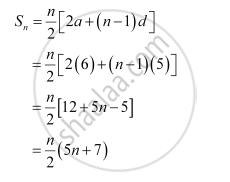Share

# Find the Sum to N Terms of the Arithmetic Progression, Whose Kth Term is 5k + 1. - Mathematics

#### Question

Find the sum to n terms of the A.P., whose kth term is 5k + 1.

#### Solution

It is given that the kth term of the A.P. is 5k + 1.

kth term = ak = + (k – 1)d

∴ + (k – 1)d = 5k + 1

a + kd – d = 5k + 1

Comparing the coefficient of k, we obtain d = 5

– = 1

⇒ a – 5 = 1

⇒ a = 6Is there an error in this question or solution?